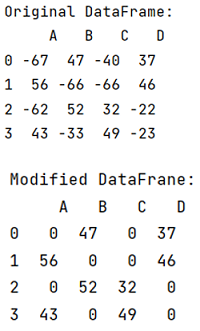# How to replace negative numbers in Pandas Data Frame by zero?

Given a Pandas DataFrame, we have to replace negative numbers by zero.
Submitted by Pranit Sharma, on July 07, 2022

Pandas is a special tool that allows us to perform complex manipulations of data effectively and efficiently. Inside pandas, we mostly deal with a dataset in the form of DataFrame. DataFrames are 2-dimensional data structures in pandas. DataFrames consist of rows, columns, and data.

## Problem statement

Given a Pandas DataFrame, we have to replace negative numbers by zero.

## Replacing negative numbers in Pandas Data Frame by zero

for this purpose, we will check that if any value is less than 0 we will assign 0 as its value. We will use df[df<0]=0, this code statement will check and assign 0, if the number is negative.

To work with pandas, we need to import pandas package first, below is the syntax:

```import pandas as pd
```

Let us understand with the help of an example,

## Python program to replace negative numbers in Pandas Data Frame by zero

```# Importing pandas package
import pandas as pd

# Creating a dictionary
d = {
'A':[-67,56,-62,43],
'B':[47,-66,52,-33],
'C':[-40,-66,32,49],
'D':[37,46,-22,-23]
}

# Creating a DataFrame
df = pd.DataFrame(d)

# Display original DataFrame
print("Original DataFrame:\n",df,"\n")

# Replacing negative values with 0
df[df<0]=0

# Display modified DataFrame
print("Modified DataFrane:\n",df)
```

## Output

The output of the above program is: